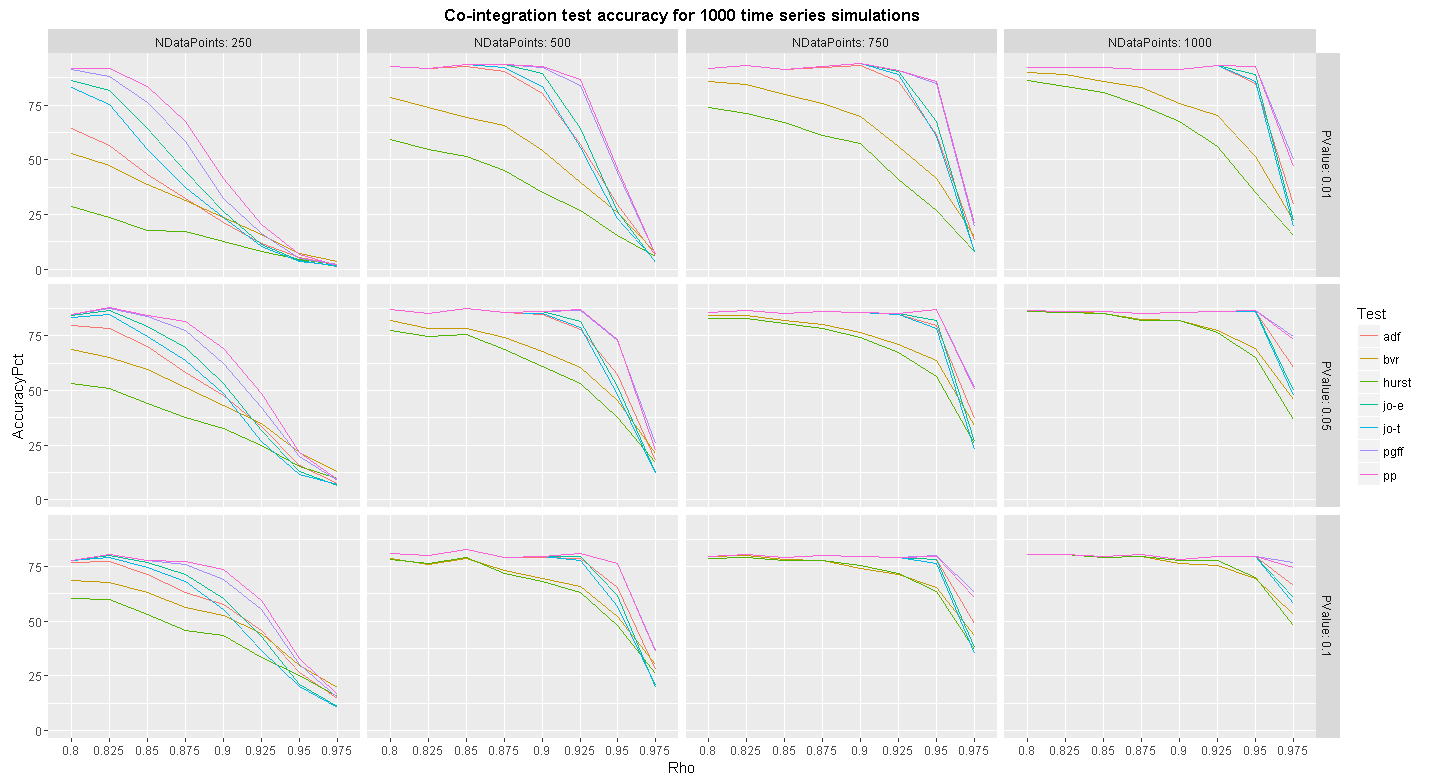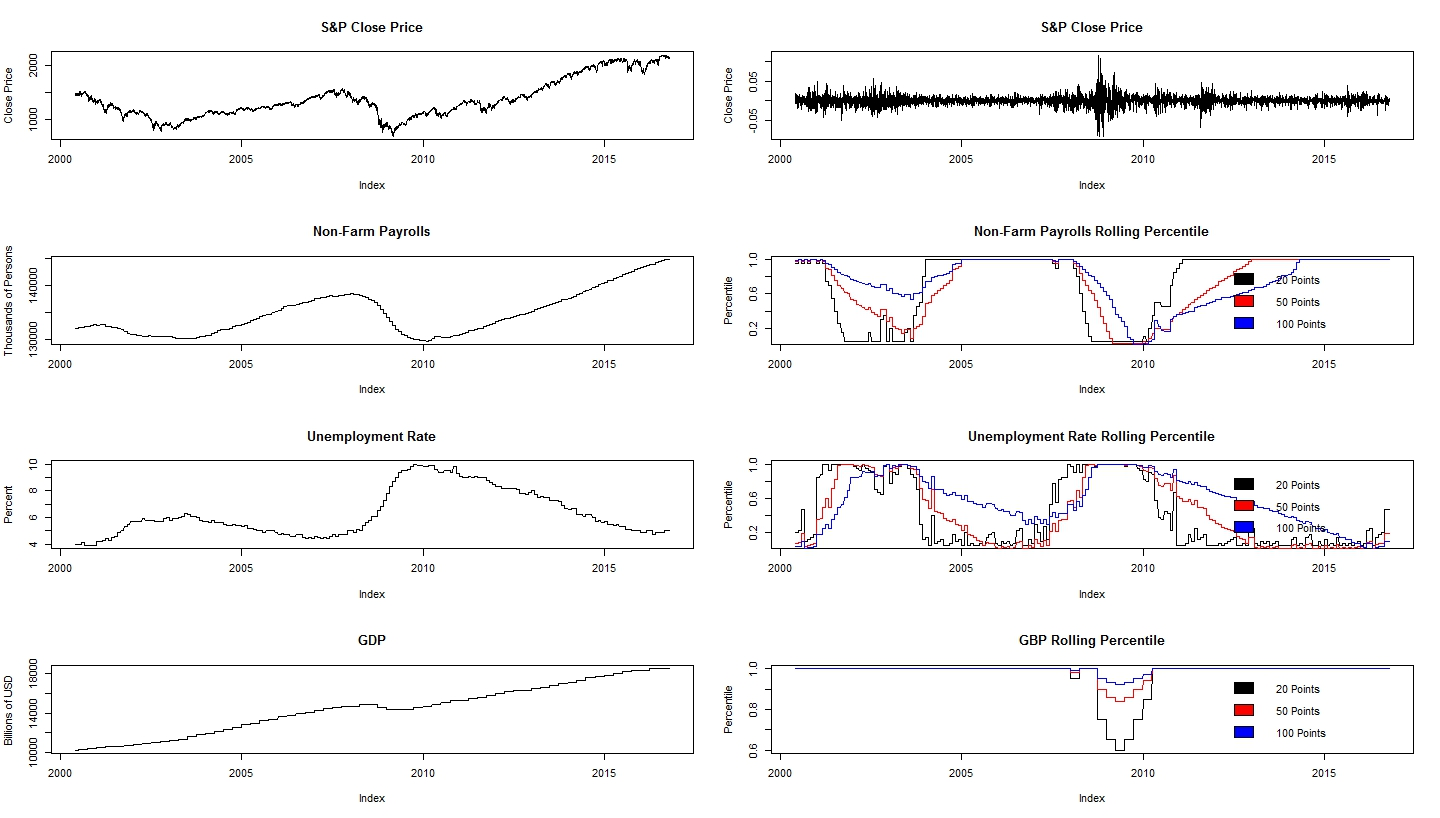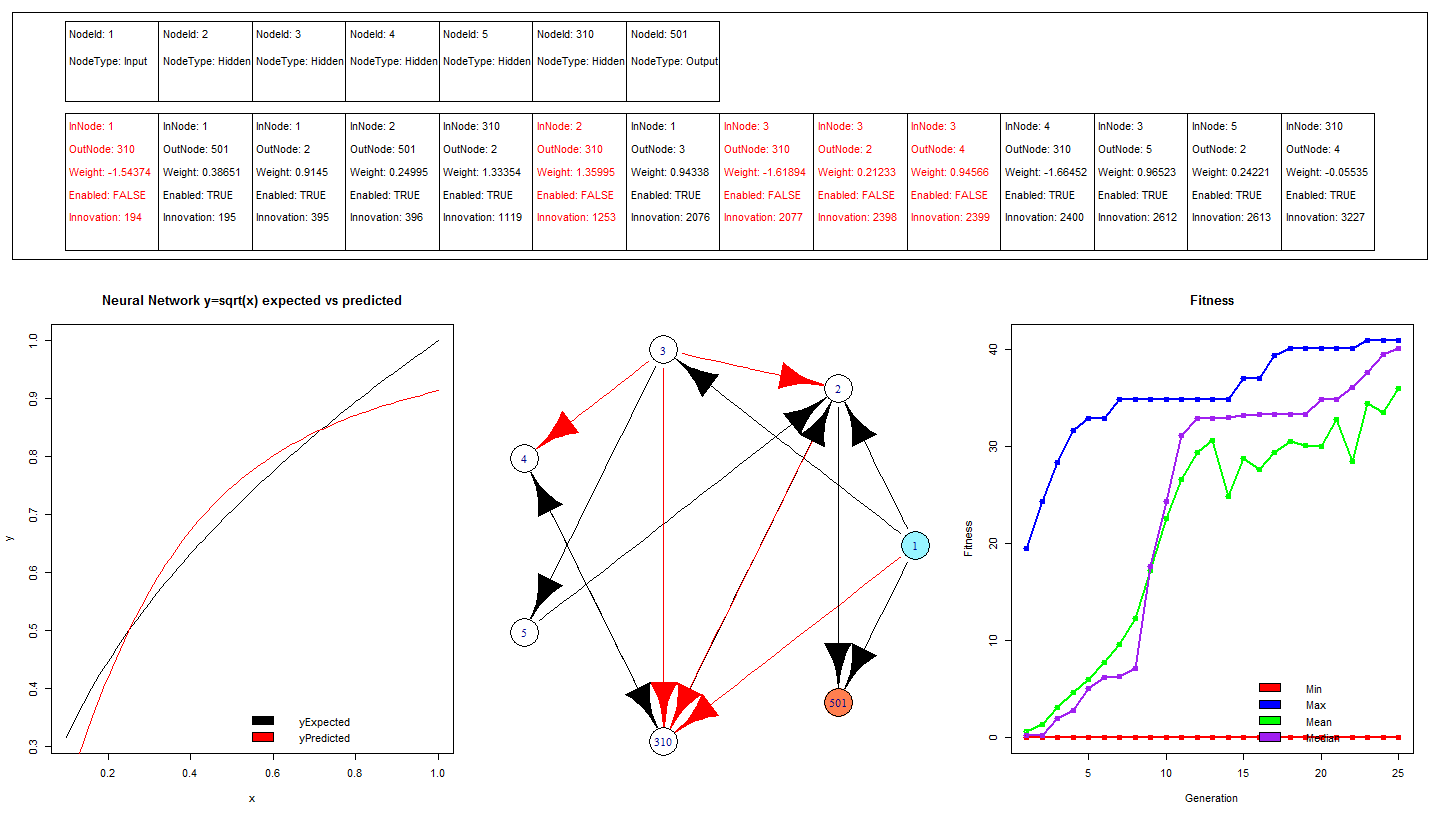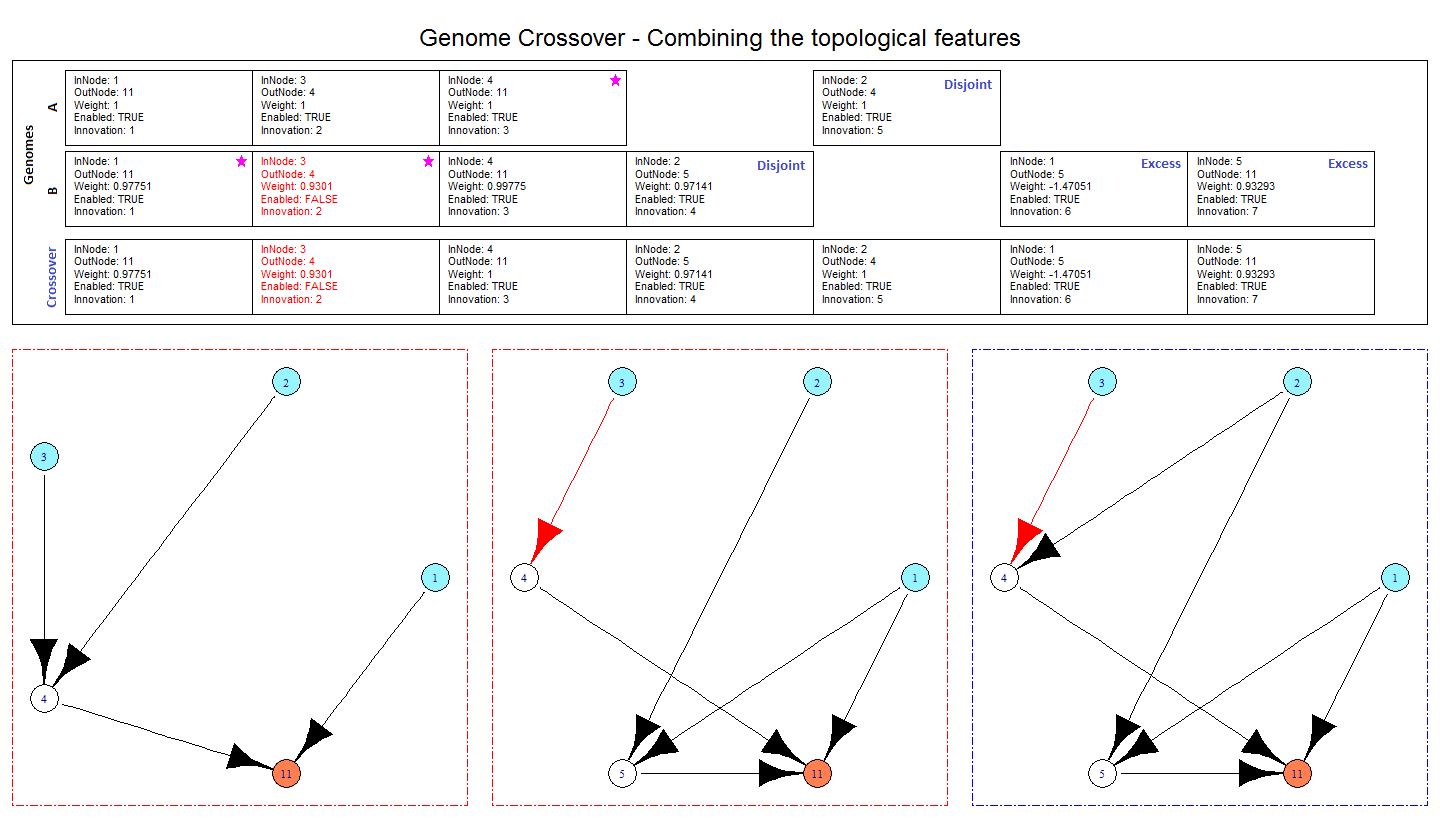# Investigation into the Power of Co-integration / mean reversion tests

The term statistical arbitrage (stat-arb) encompasses a wide variety of investment strategies that typically aim to exploit a statistical equilibrium relationship between two or more securities. The general principal is that any divergence from the equilibrium is a temporary effect and that bets should be placed on the process reverting to it’s equilibrium.

The major caveat of stat-arb /pairs trading type strategies is that as the divergence from equilibrium grows the trade becomes more desirable, however at some point the divergence will grow so large that one has to concede that the equilibrium relationship no longer exists / the model is broken. Naturally it is desirable to estimate the power of the statistical tools used to determine these relationships and to asses the duration of any observed equilibrium out of sample.

This post will investigate the power of the statistical tests in relation to pairs-trading for the following statistical tests ADF, BVR, HURST, PP, PGFF,
JO-T and JO-E.

The general principal is that for two stocks$X$ and$Y$ they form a stationary, and by definition, mean reverting pair if the following equation holds:$Y_{t} = \hat{\alpha}+\hat{\beta}X_{t}+R_{t}$$R_{t} = \hat{\rho}R_{t-1}+\epsilon_{t}$$\epsilon_{t} \sim i.i.d.N(0,\sigma^{2})$

If$\hat{\rho}$ is between$-1$ and$1$ then$X$ and$Y$ are co-integrated,$\rho$
is the coefficient of mean reversion. A statistical test must be performed to check if$\hat{\rho}=1$, this is known as a unit root test. If the series contains a unit root it isn’t suitable for pairs trading. There are multiple unit root tests, each running a different test on the residual process. One might be tempted to estimate the AR(1) residual model and check for$\rho=1$ using the conventional linear regression method calculating the standard t-ratio. However it was shown by Dicky and Fuller  that the t-ratio does not follow the t-distribution, hence non-standard significance tests are needed known as unit root tests.

As with every model there are trade off when determining the training window size, too long a window and the model may contain irrelevant data and be slow to adjust to recent events, too short a window and the model only responds to recent events and forgets about past events quickly. This trade off is problematic in co-integration testing, it was demonstrated in Clegg, M., January 2014. On the persistence of cointegration in pairs trading that for a fixed window size the power of most unit root tests decrease as as$\rho$
tends to 1 from below, for 250 data points with$\rho=0.96$
the barrage of co-integration tests only detect co-integration less than 25% of the time!

Intuitively this makes sense, the slower the process is to revert the more data points will be needed to see the reversion. It is somewhat undesirable that the power of the unit root tests vary depending upon the properties of the underlying process, however it is not required for successful pairs trading that all co-integrated pairs are identified as such the varying power property of unit root tests is largely irrelevant.

What is more interesting is the false positive rate, so pairs identified as mean reverting when they are not, and how persistent the results are.

Accuracy Test

Generate 1000 co-integrated time series with$\alpha$ and$\beta$ uniformly distributed in the set$[-10,10]$,  and$\rho$ in the set$[0.800,0.825,0.850,0.875,0.900,0.925,0.950,0.975]$ according to Clegg this is similar to the types of stock pairs encountered in reality. Repeat this for different lengths of time series and test to see how many time series get correctly classified as co-integrated / mean reverting using various tests for different pValues.In the majority of tests PP and PGFF outperform the other methods. When the process was strongly reverting with$\rho$ less than 0.85 the tests PP, PGFF, JO-E and JO-T correctly identified the process as co-integrated / mean reverting more than 75% of the time at pValue 0.01. For some of the weaker reverting pairs with$\rho$ greater than 0.95 the performance of the statistical tests is woeful with just 250 data points.

It is worth bearing in mind that 250 data points is approximatlythe number of trading days in a year, and perhaps gives an indication of how much historical data is needed in a pairs trading strategy.

False Positive Tests

Follow the same procedure outlined for the accuracy test but chose$\rho$ in the set$[1.001,1.026,1.051,1.076,1.101,1.126,1.151,1.176]$ to generate time series that isn’t co-integrated. See what percentage of the paths get falsely reported as co-integrated / mean reverting.I’ve never seen this chart in a text book and was surprised at the results, both HURST and BVR report more false positives as$\rho$ increases! The more the process explodes the more likely the test was to show a false positive!

Thankfully the other tests behave in a reasonable fashion with few false positives.

Onto the code:

?View Code RSPLUS
 library("egcm") #Has lots of co-integration tests library("ggplot2") #Used for plotting data     set.seed(1) #Makes the random number generator start from the same point for everyone so hopefully creates the same results   assertTrueFunc <- function(testExpression, messageIfNotTrue){ #Utility method to ensure parameters into other functions are correct if(!testExpression){ cat(paste("Assertion Fail:",messageIfNotTrue,"\n")) stop() } }   createTimeSeriesForRho <- function(rho,nDataPoint){ #The library egcm contains a function rcoint that generates co-integrated data: # function (n, alpha = runif(1, -10, 10), beta = runif(1, -10, 10), rho = runif(1, 0, 1), sd_eps = 1, sd_delta = 1, X0 = 0, Y0 = 0) return (rcoint(nDataPoint,rho=rho)) }   createTimeSeriesForRhoAndTestForCointegration <- function(rhos, pValues, testMethods, nDataPoints,nMonteCarlo){ combinations <- length(rhos)*length(pValues)*length(testMethods)*length(nDataPoints)*nMonteCarlo results <- data.frame(Rho=numeric(combinations),PValue=numeric(combinations),NDataPoint=numeric(combinations),Test=character(combinations),IsCointegrated=numeric(combinations),stringsAsFactors=F) counter <- 1 for(nDataPoint in nDataPoints){ for(rho in rhos){ print(paste("Progress:", round(100*counter/combinations,2),"%")) for(nMc in seq(1,nMonteCarlo)){ simData <- createTimeSeriesForRho(rho,nDataPoint) for(testMethod in testMethods){ for(pValue in pValues){     isCointegrated <- 0 try({ e <- egcm(simData,p.value=pValue,urtest=testMethod) isCointegrated<-is.cointegrated(e)*1   },silent=TRUE) results[counter,]<-c(rho,pValue,nDataPoint,testMethod,isCointegrated) counter <- counter + 1 } } } } } return (results) }   testPowerForCointegratedSeries <- function(rhos, pValues, testMethods, nDataPoints,nMonteCarlo){ assertTrueFunc(max(rhos)<1,"Rho must be less than 1") assertTrueFunc(min(rhos)>-1,"Rho must be greater than -1") assertTrueFunc(max(pValues)<1,"pValue must be less than 1") assertTrueFunc(min(pValues)>0,"pValue must be greater than 0") assertTrueFunc(min(nDataPoints)>0,"nDataPoint must be greater than 0") assertTrueFunc(nMonteCarlo>0,"nMonteCarlo must be greater than 0")   results <- createTimeSeriesForRhoAndTestForCointegration(rhos,pValues,testMethods,nDataPoints,nMonteCarlo) results <- aggregate(IsCointegrated~Rho+PValue+NDataPoint+Test,data=results,FUN=function(x){100*mean(as.numeric(x))}) colnames(results) <- c("Rho","PValue","NDataPoint","Test","AccuracyPct") return (results) }   testFalsePositiveRateForNoneCointegratedSeries <- function(rhos, pValues, testMethods, nDataPoints,nMonteCarlo){ assertTrueFunc(min(rhos)>1,"Rho must be greater than 1") assertTrueFunc(max(pValues)<1,"pValue must be less than 1") assertTrueFunc(min(pValues)>0,"pValue must be greater than 0") assertTrueFunc(min(nDataPoints)>0,"nDataPoint must be greater than 0") assertTrueFunc(nMonteCarlo>0,"nMonteCarlo must be greater than 0")   results <- createTimeSeriesForRhoAndTestForCointegration(rhos,pValues,testMethods,nDataPoints,nMonteCarlo) results <- aggregate(IsCointegrated~Rho+PValue+NDataPoint+Test,data=results,FUN=function(x){100*mean(as.numeric(x))}) colnames(results) <- c("Rho","PValue","NDataPoint","Test","FalsePositiveRatePct") return (results) }   nMC <- 1000   rhosCoint <- seq(0.8,0.999,0.025) rhosFalsePositive <- seq(1.001,1.2,0.025) nDataPoints <- c(250,500,750,1000) pValues <- c(0.01,0.05, 0.1) urTests <- c("adf","bvr","hurst","jo-e","jo-t","pp","pgff")   tt <- testPowerForCointegratedSeries(rhosCoint,pValues,urTests,nDataPoints,nMC) dev.new() tt$NDataPoints <- factor(tt$NDataPoint,levels=nDataPoints) #Creating a factor to get the chart order in same order as nDataPoints variable ggplot(tt, aes(Rho,AccuracyPct,color=Test))+ geom_line(aes(group=Test))+ facet_grid(PValue ~ NDataPoints,labeller = label_both)+ ggtitle(paste("Co-integration test accuracy for",nMC,"time series simulations"))+ theme(plot.title = element_text(lineheight=.8, face="bold"))   qq <- testFalsePositiveRateForNoneCointegratedSeries(rhosFalsePositive,pValues,urTests,nDataPoints,nMC)   dev.new() qq$NDataPoints <- factor(qq$NDataPoint,levels=nDataPoints) #Creating a factor to get the chart order in same order as nDataPoints variable ggplot(qq, aes(Rho,FalsePositiveRatePct,color=Test))+ geom_line(aes(group=Test))+ facet_grid(PValue ~ NDataPoints,labeller = label_both)+ ggtitle(paste("Co-integration test false positive rate for",nMC,"time series simulations"))+ theme(plot.title = element_text(lineheight=.8, face="bold"))

# Evolving Neural Networks through Augmenting Topologies – Part 4 of 4 – Trading Strategy

This post explores applying NEAT to trading the S&P. The learned strategy significantly out performs buying and holding both in and out of sample.

Features:

A key part of any machine learning problem is defining the features and ensuring that they’re normalised in some fashion.
The features will be rolling percentiles of the following economic data, a rolling percentile takes the last n data points and calculates what % of data point the latest data point is greater than.

• Non-farm payrolls
• Unemployment Rate
• GDP

Fitness Function

The fitness function is final equity, and aims to maximise the final equity

Termination Function

Any genome that has a 20% draw down, or attempts to use a leverage greater than +/- 2 is terminated. In practise you wouldn’t want to make your system machine learn the risk controls as there is potential that they don’t get learned. The reason they are embedded inside the strategy is to speed up the learning process as we can kill genomes early before the simulation is complete based upon breaking the risk rules.

Plot of all data / features

It appears that when non-farms fall to their lower percentiles / unemployment reaches it’s highest percentiles the day to day returns in the S&P become more volatile. It is hoped that the learning can take advantage of this.Training Results

The learning has identified a strategy that out performs simply buying and holding. The proposed strategy has a max drawdown around 20% vs the buy and hold having a draw down of 40%. Additionally the strategy shorted the index between 2000-2003 as it was selling off before going long to 2007. Generating a return of 80% vs buy and hold of 7%!

Out of sample results

In the out of sample data (not used during the training) the strategy significantly out performed buying and holding, approx 250% return vs 50% with a max drawdown close to 20% vs buy and hold draw down of 50%.Onto the code:

?View Code RSPLUS
 install.packages("devtools") library("devtools") install_github("RNeat","ahunteruk") #Install from github as not yet on CRAN library("RNeat") library("quantmod")   marketSymbol <- "^GSPC" econmicDataSymbols <- c("UNRATE","PAYEMS","GDP")   mktData <- new.env() #Make a new environment for quantmod to store data in economicData <- new.env() #Make a new environment for quantmod to store data in   #Specify dates for downloading data, training models and running simulation dataDownloadStartDate <- as.Date("2000-06-01")   trainingStartDate = as.Date("2001-01-01") #Specify the date to start training (yyyy-mm-dd) trainingEndDate = as.Date("2006-12-31") #Specify the date to end training   outOfSampleStartDate = as.Date("2007-01-01") outOfSampleEndDate = as.Date("2016-07-15")   #Download Data getSymbols(marketSymbol,env=mktData,from=dataDownloadStartDate) #S&P 500 getSymbols(econmicDataSymbols,src="FRED",env=economicData,from=dataDownloadStartDate) #Payems is non-farms payrolls   nEconomicDataPercentileLookbackShort <- 20 nEconomicDataPercentileLookbackMedium <- 50 nEconomicDataPercentileLookbackLong <- 100   rollingPercentile <- function(data,n){ percentile <- function(dataBlock){ last(rank(dataBlock)/length(dataBlock)) } return (as.zoo(rollapply(as.zoo(data),width=n,percentile,align="right",by.column=TRUE))) }   stockCleanNameFunc <- function(name){ return(sub("^","",name,fixed=TRUE)) }   clClRet <- as.zoo((lag(Cl(get(stockCleanNameFunc(marketSymbol),mktData)),-1)/Cl(get(stockCleanNameFunc(marketSymbol),mktData))-1))   payemsShortPercentile <- rollingPercentile(economicData$PAYEMS,nEconomicDataPercentileLookbackShort) payemsMediumPercentile <- rollingPercentile(economicData$PAYEMS,nEconomicDataPercentileLookbackMedium) payemsLongPercentile <- rollingPercentile(economicData$PAYEMS,nEconomicDataPercentileLookbackLong) unrateShortPercentile <- rollingPercentile(economicData$UNRATE,nEconomicDataPercentileLookbackShort) unrateMediumPercentile <- rollingPercentile(economicData$UNRATE,nEconomicDataPercentileLookbackMedium) unrateLongPercentile <- rollingPercentile(economicData$UNRATE,nEconomicDataPercentileLookbackLong)   gdpShortPercentile <- rollingPercentile(economicData$GDP,nEconomicDataPercentileLookbackShort) gdpMediumPercentile <- rollingPercentile(economicData$GDP,nEconomicDataPercentileLookbackMedium) gdpLongPercentile <- rollingPercentile(economicData$GDP,nEconomicDataPercentileLookbackLong) #join the data sets, fill in any missing dates with the previous none NA value mergedData <- na.locf(merge(economicData$PAYEMS,merge(Cl(get(stockCleanNameFunc(marketSymbol),mktData)), economicData$PAYEMS,payemsShortPercentile,payemsMediumPercentile,payemsLongPercentile, economicData$UNRATE,unrateShortPercentile,unrateMediumPercentile,unrateLongPercentile, economicData$GDP,gdpShortPercentile,gdpMediumPercentile,gdpLongPercentile ,all.x=T),all=T)) mergedData <- mergedData[,-1] ClClRet <- as.zoo(lag(mergedData[,1],-1)/mergedData[,1]-1) ClTZero <- as.zoo(mergedData[,1]) ClTOne <- as.zoo(lag(mergedData[,1],-1)) mergedData <- merge(ClClRet,ClTOne,ClTZero,mergedData) mergedData <- window(mergedData,start=dataDownloadStartDate) colnames(mergedData) <- c("ClClRet","ClTOne","ClTZero","Price","Payems","Payems.short","Payems.medium","Payems.long", "Unrate","Unrate.short","Unrate.medium","Unrate.long", "Gdp","Gdp.short","Gdp.medium","Gdp.long","all.x") dev.new() par(mfrow=c(4,2)) plot(mergedData[,"Price"], main="S&P Close Price",ylab="Close Price") plot(mergedData[,"ClClRet"], main="S&P Close Price",ylab="Close Price") plot(mergedData[,"Payems"], main="Non-Farm Payrolls",ylab="Thousands of Persons") plot(mergedData[,"Payems.short"], main="Non-Farm Payrolls Rolling Percentile",ylab="Percentile") lines(mergedData[,"Payems.medium"], col="red") lines(mergedData[,"Payems.long"], col="blue") legend(x='bottomright', c(paste(nEconomicDataPercentileLookbackShort,"Points"), paste(nEconomicDataPercentileLookbackMedium,"Points"), paste(nEconomicDataPercentileLookbackLong,"Points")), fill=c("black","red","blue"), bty='n') plot(mergedData[,"Unrate"], main="Unemployment Rate",ylab="Percent") plot(mergedData[,"Unrate.short"], main="Unemployment Rate Rolling Percentile",ylab="Percentile") lines(mergedData[,"Unrate.medium"], col="red") lines(mergedData[,"Unrate.long"], col="blue") legend(x='bottomright', c(paste(nEconomicDataPercentileLookbackShort,"Points"), paste(nEconomicDataPercentileLookbackMedium,"Points"), paste(nEconomicDataPercentileLookbackLong,"Points")), fill=c("black","red","blue"), bty='n') plot(mergedData[,"Gdp"], main="GDP",ylab="Billions of USD") plot(mergedData[,"Gdp.short"], main="GBP Rolling Percentile",ylab="Percentile") lines(mergedData[,"Gdp.medium"], col="red") lines(mergedData[,"Gdp.long"], col="blue") legend(x='bottomright', c(paste(nEconomicDataPercentileLookbackShort,"Points"), paste(nEconomicDataPercentileLookbackMedium,"Points"), paste(nEconomicDataPercentileLookbackLong,"Points")), fill=c("black","red","blue"), bty='n') featuresTrainingData <- window(mergedData,start=trainingStartDate,end=trainingEndDate) featuresOutOfSampleData <- window(mergedData,start=outOfSampleStartDate,end=outOfSampleEndDate) #Genetic algo setup simulationData <- featuresTrainingData trading.InitialState <- function(){ state <- list() state <- 100 #Equity state <- 0 #% of Equity allocated to share (-ve for shorts) state <- state #Maximum equity achieved state <- 1 #Trading day number state <- simulationData[1,"Price"] state <- simulationData[1,"Payems.short"] state <- simulationData[1,"Payems.medium"] state <- simulationData[1,"Payems.long"] state <- simulationData[1,"Unrate.short"] state <- simulationData[1,"Unrate.medium"] state <- simulationData[1,"Unrate.long"] state <- simulationData[1,"Gdp.short"] state <- simulationData[1,"Gdp.medium"] state <- simulationData[1,"Gdp.long"] return(state) } trading.ConvertStateToNeuralNetInputs <- function(currentState){ return (currentState) } trading.UpdateState <- function(currentState,neuralNetOutputs){ #print(currentState) equity <- currentState[] equityAllocation <- neuralNetOutputs[] maxEquityAchieved <- currentState[] tradingDay <- currentState[] pctChange <- as.double((simulationData[tradingDay+1,"Price"]))/as.double((simulationData[tradingDay,"Price"]))-1 #print(paste("pctChange",pctChange)) #print(paste("equityAllocation",equityAllocation)) pnl <- equity * equityAllocation * pctChange equity <- equity + pnl maxEquityAchieved <- max(maxEquityAchieved,equity) tradingDay <- tradingDay + 1 currentState <- equity currentState <- equityAllocation currentState <- maxEquityAchieved currentState <- tradingDay currentState <- simulationData[tradingDay,"Price"] currentState <- simulationData[tradingDay,"Payems.short"] currentState <- simulationData[tradingDay,"Payems.medium"] currentState <- simulationData[tradingDay,"Payems.long"] currentState <- simulationData[tradingDay,"Unrate.short"] currentState <- simulationData[tradingDay,"Unrate.medium"] currentState <- simulationData[tradingDay,"Unrate.long"] currentState <- simulationData[tradingDay,"Gdp.short"] currentState <- simulationData[tradingDay,"Gdp.medium"] currentState <- simulationData[tradingDay,"Gdp.long"] return (currentState) } trading.UpdateFitness <- function(oldState,updatedState,oldFitness){ return (as.double(updatedState)) #equity achieved } trading.CheckForTermination <- function(frameNum,oldState,updatedState,oldFitness,newFitness){ equity <- updatedState[] equityAllocation <- updatedState[] maxEquityAchieved <- updatedState[] tradingDay <- updatedState[] if(tradingDay >= nrow(simulationData)){ return(T) } if(abs(equityAllocation) > 2){ #Too much leverage return(T) } if(equity/maxEquityAchieved < 0.8){ #20% draw down return(T) } else { return (F) } } trading.PlotState <-function(updatedState){ equity <- currentState[] equityAllocation <- currentState[] maxEquityAchieved <- currentState[] plot(updatedState) } plotStateAndInputDataFunc <- function(stateData, inputData, titleText){ buyandholdret <- inputData[,"Price"]/coredata(inputData[1,"Price"]) strategyret <- stateData[,"Equity"]/100 maxbuyandholdret <- cummax(buyandholdret) buyandholddrawdown <- (buyandholdret/maxbuyandholdret-1) strategydrawdown <- (stateData[,"Equity"]/stateData[,"MaxEquity"]-1) dev.new() par(mfrow=c(4,2),oma = c(0, 0, 2, 0)) plot(stateData[,"Price"],main="Price",ylab="Price") plot(buyandholdret,main="Performance (Return on Initial Equity)", ylab="Return", ylim=c(min(buyandholdret,strategyret),max(buyandholdret,strategyret))) lines(strategyret,col="red") legend(x='bottomright', c('Buy & Hold','Strategy'), fill=c("black","red"), bty='n') plot(inputData[,"ClClRet"],main="Stock Returns", ylab="Return") plot(maxbuyandholdret*100,main="Max Equity", ylim=c(min(maxbuyandholdret*100,stateData[,"MaxEquity"]),max(maxbuyandholdret*100,stateData[,"MaxEquity"])),ylab="Equity$") lines(stateData[,"MaxEquity"],col="red") legend(x='bottomright', c('Buy & Hold','Strategy'), fill=c("black","red"), bty='n') plot(inputData[,"Payems.short"], main="Payrolls Rolling Percentile",ylab="Percentile") lines(inputData[,"Payems.medium"], col="red") lines(inputData[,"Payems.long"], col="blue") legend(x='bottomright', c(paste(nEconomicDataPercentileLookbackShort,"Points"), paste(nEconomicDataPercentileLookbackMedium,"Points"), paste(nEconomicDataPercentileLookbackLong,"Points")), fill=c("black","red","blue"), bty='n')   plot(buyandholddrawdown,main="Draw Down",ylab="Percent (%)") lines(strategydrawdown,col="red") legend(x='bottomright', c('Buy & Hold','Strategy'), fill=c("black","red"), bty='n') plot(stateData[,"Allocation"],main="Allocation",ylab="Allocation")     mtext(titleText, outer = TRUE, cex = 1.5) }     config <- newConfigNEAT(14,1,500,50) tradingSimulation <- newNEATSimulation(config, trading.InitialState, trading.UpdateState, trading.ConvertStateToNeuralNetInputs, trading.UpdateFitness, trading.CheckForTermination, trading.PlotState)   tradingSimulation <- NEATSimulation.RunSingleGeneration(tradingSimulation)   for(i in seq(1,35)){ save.image(file="tradingSim.RData") #So we can recover if we crash for any reason tradingSimulation <- NEATSimulation.RunSingleGeneration(tradingSimulation) }   dev.new() plot(tradingSimulation)   stateHist <- NEATSimulation.GetStateHistoryForGenomeAndSpecies(tradingSimulation)     colnames(stateHist) <- c("Equity","Allocation","MaxEquity","TradingDay","Price", "Payems.short","Payems.medium","Payems.long", "Unrate.short","Unrate.medium","Unrate.long", "Gdp.short","Gdp.medium","Gdp.long")   row.names(stateHist)<-row.names(as.data.frame(simulationData[1:nrow(stateHist),])) stateHist <- as.zoo(stateHist) plotStateAndInputDataFunc(stateHist,simulationData,"Training Data")     simulationData <- featuresOutOfSampleData stateHist <- NEATSimulation.GetStateHistoryForGenomeAndSpecies(tradingSimulation)   colnames(stateHist) <- c("Equity","Allocation","MaxEquity","TradingDay","Price", "Payems.short","Payems.medium","Payems.long", "Unrate.short","Unrate.medium","Unrate.long", "Gdp.short","Gdp.medium","Gdp.long")   row.names(stateHist)<-row.names(as.data.frame(simulationData[1:nrow(stateHist),])) stateHist <- as.zoo(stateHist)   plotStateAndInputDataFunc(stateHist,simulationData,"Out of Sample Data")

# RNeat – Square Root Neural Net trained using Augmenting Topologies – Simple Example

A simple tutorial demonstrating how to train a neural network to square root numbers using a genetic algorithm that searches through the topological structure space. The algorithm is called NEAT (Neuro Evolution of Augmenting Topologies) available in the RNeat package (not yet on CRAN).

The training is very similar to other machine learning / regression packages in R. The training function takes a data frame and a formula. The formula is used to specify what columns in the data frame are the dependent variables and which are the explanatory variable. The code is commented and should be simple enough for new R users.The performance of the network can be seen in the bottom left chart of the image above, there is considerable differences between the expected output and the actual output. It is likely that with more training the magnitude of these errors will reduce, it can be seen in the bottom right chart that the maximum, mean and median fitness are generally increasing with each generation.

?View Code RSPLUS
 install.packages("devtools") library("devtools") install_github("RNeat","ahunteruk") #Install from github as not yet on CRAN library("RNeat")   #Generate traing data y = sqrt(x) trainingData <- as.data.frame(cbind(sqrt(seq(0.1,1,0.1)),seq(0.1,1,0.1))) colnames(trainingData) <- c("y","x")   #Train the neural network for 5 generations, and plot the fitness rneatsim <- rneatneuralnet(y~x,trainingData,5) plot(rneatsim)   #Continue training the network for another 5 generations rneatsim <- rneatneuralnetcontinuetraining(rneatsim,20) plot(rneatsim)   #Construct some fresh data to stick through the neural network and hopefully get square rooted liveData <- as.data.frame(seq(0.1,1,0.01)) colnames(liveData) <- c("x")   liveDataExpectedOutput <- sqrt(liveData) colnames(liveDataExpectedOutput) <- "yExpected"   #Pass the data through the network results <- compute(rneatsim,liveData)   #Calculate the difference between yPred the neural network output, and yExpected the actual square root of the input error <- liveDataExpectedOutput[,"yExpected"] - results[,"yPred"] results <- cbind(results,liveDataExpectedOutput,error)   dev.new() layout(matrix(c(3,3,3,1,4,2), 2, 3, byrow = TRUE),heights=c(1,2)) plot(x=results[,"x"],y=results[,"yExpected"],type="l", main="Neural Network y=sqrt(x) expected vs predicted",xlab="x",ylab="y") lines(x=results[,"x"],y=results[,"yPred"],col="red",type="l") legend(x='bottomright', c('yExpected','yPredicted'), col=c("black","red"), fill=1:2, bty='n') plot(rneatsim) plot(rneatsim\$simulation)

# Evolving Neural Networks through Augmenting Topologies – Part 3 of 4

This part of the NEAT tutorial will show how to use the RNeat package (not yet on CRAN) to solve the classic pole balance problem.

The simulation requires the implementation of 5 functions:

• processInitialStateFunc – This specifies the initial state of the system, for the pole balance problem the state is the cart location, cart velocity, cart acceleration, force being applied to the cart, pole angle, pole angular velocity and pole angular acceleration.
• processUpdateStateFunc – This specifies how to take the current state and update it using the outputs of the neural network. In this example this function simulates the equations of motion and takes the neural net output as the force that is being applied to the cart.
• processStateToNeuralInputFunc – Allows for modifying the state / normalisation of the state before it is passed as an input to the neural network
• fitnessUpdateFunc – Takes the old fitness, the old state and the new updated state and determines what the new system fitness is. For the pole balance problem this function wants to reward the pendulum being up right, and reward the cart being close to the middle of the track.
• terminationCheckFunc – Takes the state and checks to see if the termination should be terminated. Can chose to terminate if the pole falls over, the simulation has ran too long or the cart has driven off of the end of the track.
• plotStateFunc – Plots the state, for the pole balance this draws the cart and pendulum.

Onto the code:

?View Code RSPLUS
 install.packages("devtools") library("devtools") install_github("RNeat","ahunteruk") #Install from github as not yet on CRAN library("RNeat") drawPoleFunc <- function(fixedEnd.x,fixedEnd.y,poleLength, theta,fillColour=NA, borderColour="black"){ floatingEnd.x <- fixedEnd.x-poleLength * sin(theta) floatingEnd.y <- fixedEnd.y+poleLength * cos(theta)   polygon(c(fixedEnd.x,floatingEnd.x,floatingEnd.x,fixedEnd.x), c(fixedEnd.y,floatingEnd.y,floatingEnd.y,fixedEnd.y), col = fillColour, border=borderColour) }   drawPendulum <- function(fixedEnd.x,fixedEnd.y,poleLength, theta,radius,fillColour=NA, borderColour="black"){ floatingEnd.x <- fixedEnd.x-poleLength * sin(theta) floatingEnd.y <- fixedEnd.y+poleLength * cos(theta) createCircleFunc(floatingEnd.x,floatingEnd.y,radius,fillColour,borderColour) }   #Parameters to control the simulation simulation.timestep = 0.005 simulation.gravity = 9.8 #meters per second^2 simulation.numoftimesteps = 2000   pole.length = 1 #meters, total pole length pole.width = 0.2 pole.theta = pi/4 pole.thetaDot = 0 pole.thetaDotDot = 0 pole.colour = "purple"     pendulum.centerX = NA pendulum.centerY = NA pendulum.radius = 0.1 pendulum.mass = 0.1 pendulum.colour = "purple"   cart.width=0.5 cart.centerX = 0 cart.centerY = 0 cart.height=0.2 cart.colour="red" cart.centerXDot = 0 cart.centerXDotDot = 0 cart.mass = 0.4 cart.force = 0 cart.mu=2     track.limit= 10 #meters from center track.x = -track.limit track.height=0.01 track.y = 0.5*track.height track.colour = "blue"   leftBuffer.width=0.1 leftBuffer.height=0.2 leftBuffer.x=-track.limit-0.5*cart.width-leftBuffer.width leftBuffer.y=0.5*leftBuffer.height leftBuffer.colour = "blue"   rightBuffer.width=0.1 rightBuffer.height=0.2 rightBuffer.x=track.limit+0.5*cart.width rightBuffer.y=0.5*rightBuffer.height rightBuffer.colour = "blue"   #Define the size of the scene (used to visualise what is happening in the simulation) scene.width = 2*max(rightBuffer.x+rightBuffer.width,track.limit+pole.length+pendulum.radius) scene.bottomLeftX = -0.5*scene.width scene.height=max(pole.length+pendulum.radius,scene.width) scene.bottomLeftY = -0.5*scene.height   poleBalance.InitialState <- function(){ state <- list() state <- cart.centerX state <- cart.centerXDot state <- cart.centerXDotDot state <- cart.force state <- pole.theta state <- pole.thetaDot state <- pole.thetaDotDot return(state) }   poleBalance.ConvertStateToNeuralNetInputs <- function(currentState){ return (currentState) }   poleBalance.UpdatePoleState <- function(currentState,neuralNetOutputs){ #print("Updating pole state") #print(neuralNetOutputs) cart.centerX <- currentState[] cart.centerXDot <- currentState[] cart.centerXDotDot <- currentState[] cart.force <- currentState[]+neuralNetOutputs[] pole.theta <- currentState[] pole.thetaDot <- currentState[] pole.thetaDotDot <- currentState[]   costheta = cos(pole.theta) sintheta = sin(pole.theta) totalmass = cart.mass+pendulum.mass masslength = pendulum.mass*pole.length   pole.thetaDotDot = (simulation.gravity*totalmass*sintheta+costheta*(cart.force-masslength*pole.thetaDot^2*sintheta-cart.mu*cart.centerXDot))/(pole.length*(totalmass-pendulum.mass*costheta^2))   cart.centerXDotDot = (cart.force+masslength*(pole.thetaDotDot*costheta-pole.thetaDot^2*sintheta)-cart.mu*cart.centerXDot)/totalmass   cart.centerX = cart.centerX+simulation.timestep*cart.centerXDot cart.centerXDot = cart.centerXDot+simulation.timestep*cart.centerXDotDot pole.theta = (pole.theta +simulation.timestep*pole.thetaDot ) pole.thetaDot = pole.thetaDot+simulation.timestep*pole.thetaDotDot   currentState <- cart.centerX currentState <- cart.centerXDot currentState <- cart.centerXDotDot currentState <- cart.force currentState <- pole.theta currentState <- pole.thetaDot currentState <- pole.thetaDotDot return (currentState) }       poleBalance.UpdateFitness <- function(oldState,updatedState,oldFitness){ #return (oldFitness+1) #fitness is just how long we've ran for #return (oldFitness+((track.limit-abs(updatedState[]))/track.limit)^2) #More reward for staying near middle of track   height <- cos(updatedState[]) #is -ve if below track heightFitness <- max(height,0) centerFitness <- (track.limit-abs(updatedState[]))/track.limit return (oldFitness+(heightFitness + heightFitness*centerFitness)) }   poleBalance.CheckForTermination <- function(frameNum,oldState,updatedState,oldFitness,newFitness){ cart.centerX <- updatedState[] cart.centerXDot <- updatedState[] cart.centerXDotDot <- updatedState[] cart.force <- updatedState[] pole.theta <- updatedState[] pole.thetaDot <- updatedState[] pole.thetaDotDot <- updatedState[]   oldpole.theta <- oldState[] if(frameNum > 20000){ print("Max Frame Num Exceeded , stopping simulation") return (T) }   height <- cos(pole.theta) oldHeight <- cos(oldpole.theta) if(height==-1 & cart.force==0){ return(T) }   if(oldHeight >= 0 & height < 0){ #print("Pole fell over") return (T) } if(cart.centerX < track.x | cart.centerX > (track.x+2*track.limit)){ #print("Exceeded track length") return (T) } else { return (F) } }   poleBalance.PlotState <-function(updatedState){ cart.centerX <- updatedState[] cart.centerXDot <- updatedState[] cart.centerXDotDot <- updatedState[] cart.force <- updatedState[] pole.theta <- updatedState[] pole.thetaDot <- updatedState[] pole.thetaDotDot <- updatedState[]   createSceneFunc(scene.bottomLeftX,scene.bottomLeftY,scene.width,scene.height, main="Simulation of Inverted Pendulum - www.gekkoquant.com",xlab="", ylab="",xlim=c(-0.5*scene.width,0.5*scene.width),ylim=c(-0.5*scene.height,0.5*scene.height))   createBoxFunc(track.x,track.y,track.limit*2,track.height,track.colour) createBoxFunc(leftBuffer.x,leftBuffer.y,leftBuffer.width,leftBuffer.height,leftBuffer.colour) createBoxFunc(rightBuffer.x,rightBuffer.y,rightBuffer.width,rightBuffer.height,rightBuffer.colour) createBoxFunc(cart.centerX-0.5*cart.width,cart.centerY+0.5*cart.height,cart.width,cart.height,cart.colour) drawPoleFunc(cart.centerX,cart.centerY,2*pole.length,pole.theta,pole.colour) drawPendulum(cart.centerX,cart.centerY,2*pole.length,pole.theta,pendulum.radius,pendulum.colour)   }   config <- newConfigNEAT(7,1,500,50) poleSimulation <- newNEATSimulation(config, poleBalance.InitialState, poleBalance.UpdatePoleState, poleBalance.ConvertStateToNeuralNetInputs, poleBalance.UpdateFitness, poleBalance.CheckForTermination, poleBalance.PlotState)   for(i in seq(1,1000)){ poleSimulation <- NEATSimulation.RunSingleGeneration(poleSimulation,T,"videos","poleBalance",1/simulation.timestep) }

# Evolving Neural Networks through Augmenting Topologies – Part 2 of 4

This part of the tutorial on using NEAT algorithm explains how genomes are crossed over in a meaningful way maintaining their topological information and how speciation (group genomes into species) can be used to protect weak genomes with new topological information from prematurely being eradicated from the gene pool before their weight space can be optimised.

The first part of this tutorial can be found here.

Tracking Gene History through Innovation Numbers

Part 1 showed two mutations, link mutate and node mutate which both added new genes to the genome. Each time a new gene is created (through a topological innovation) a global innovation number is incremented and assigned to that gene.

The global innovation number is tracking the historical origin of each gene. If two genes have the same innovation number then they must represent the same topology (although the weights may be different). This is exploited during the gene crossover.

Genome Crossover (Mating)

Genomes crossover takes two parent genomes (lets call them A and B) and creates a new genome (lets call it the child) taking the strongest genes from A and B copying any topological structures along the way.

During the crossover genes from both genomes are lined up using their innovation number. For each innovation number the gene from the most fit parent is selected and inserted into the child genome. If both parent genomes are the same fitness then the gene is randomly selected from either parent with equal probability. If the innovation number is only present in one parent then this is known as a disjoint or excess gene and represents a topological innovation, it too is inserted into the child.

The image below shows the crossover process for two genomes of the same fitness.Speciation

Speciation takes all the genomes in a given genome pool and attempts to split them into distinct groups known as species. The genomes in each species will have similar characteristics.

A way of measuring the similarity between two genomes is required, if two genomes are “similar” they are from the same species. A natural measure to use would be a weighted sum of the number of disjoint & excess genes (representing topological differences) and the difference in weights between matching genes. If the weighted sum is below some threshold then the genomes are of the same species.

The advantage of splitting the genomes into species is that during the genetic evolution step where genomes with low fitness are culled (removed entirely from the genome pool) rather than having each genome fight for it’s place against every other genome in the entire genome pool we can make it fight for it’s place against genomes of the same species. This way species that form from a new topological innovation that might not have a high fitness yet due to not having it’s weights optimised will survive the culling.

Summary of whole process

• Create a genome pool with n random genomes
• Take each genome and apply to problem / simulation and calculate the genome fitness
• Assign each genome to a species
• In each species cull the genomes removing some of the weaker genomes
• Breed each species (randomly select genomes in the species to either crossover or mutate)
• Repeat all of the above